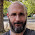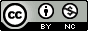## Thursday, February 26, 2015

### Using and Abusing Data Visualization: Anscombe's Quartet and Cheating Bonferroni

Anscombe’s quartet comprises four datasets that have nearly identical simple statistical properties, yet appear very different when graphed. Each dataset consists of eleven (x,y) points. They were constructed in 1973 by the statistician Francis Anscombe to demonstrate both the importance of graphing data before analyzing it and the effect of outliers on statistical properties.
Let’s load and view the data. There’s a built-in dataset, but I munged the data into a tidy format and included it in an R package that I wrote primarily for myself.
``````# If you don't have Tmisc installed, first install devtools, then install
# from github: install.packages('devtools')
# devtools::install_github('stephenturner/Tmisc')
library(Tmisc)
data(quartet)
str(quartet)
``````
``````## 'data.frame':    44 obs. of  3 variables:
##  \$ set: Factor w/ 4 levels "I","II","III",..: 1 1 1 1 1 1 1 1 1 1 ...
##  \$ x  : int  10 8 13 9 11 14 6 4 12 7 ...
##  \$ y  : num  8.04 6.95 7.58 8.81 8.33 ...
``````
set x y
I 10 8.04
I 8 6.95
I 13 7.58
II 10 9.14
II 8 8.14
II 13 8.74
III 10 7.46
III 8 6.77
III 13 12.74
IV 8 6.58
IV 8 5.76
IV 8 7.71
Now, let’s compute the mean and standard deviation of both x and y, and the correlation coefficient between x and y for each dataset.
``````library(dplyr)
quartet %>%
group_by(set) %>%
summarize(mean(x), sd(x), mean(y), sd(y), cor(x,y))
``````
``````## Source: local data frame [4 x 6]
##
##   set mean(x) sd(x) mean(y) sd(y) cor(x, y)
## 1   I       9  3.32     7.5  2.03     0.816
## 2  II       9  3.32     7.5  2.03     0.816
## 3 III       9  3.32     7.5  2.03     0.816
## 4  IV       9  3.32     7.5  2.03     0.817
``````
Looks like each dataset has the same mean, median, standard deviation, and correlation coefficient between x and y.
Now, let’s plot y versus x for each set with a linear regression trendline displayed on each plot:
``````library(ggplot2)
p = ggplot(quartet, aes(x, y)) + geom_point()
p = p + geom_smooth(method = lm, se = FALSE)
p = p + facet_wrap(~set)
p
``````

This classic example really illustrates the importance of looking at your data, not just the summary statistics and model parameters you compute from it.
With that said, you can’t use data visualization to “cheat” your way into statistical significance. I recently had a collaborator who wanted some help automating a data visualization task so that she could decide which correlations to test. This is a terrible idea, and it’s going to get you in serious type I error trouble. To see what I mean, consider an experiment where you have a single outcome and lots of potential predictors to test individually. For example, some outcome and a bunch of SNPs or gene expression measurements. You can’t just visually inspect all those relationships then cherry-pick the ones you want to evaluate with a statistical hypothesis test, thinking that you’ve outsmarted your way around a painful multiple-testing correction.
Here’s a simple simulation showing why that doesn’t fly. In this example, I’m simulating 100 samples with a single outcome variable y and 64 different predictor variables, x. I might be interested in which x variable is associated with my y (e.g., which of my many gene expression measurement is associated with measured liver toxicity). But in this case, both x and y are random numbers. That is, I know for a fact the null hypothesis is true, because that’s what I’ve simulated. Now we can make a scatterplot for each predictor variable against our outcome, and look at that plot.
``````library(dplyr)
set.seed(42)
ndset = 64
n = 100
d = data_frame(
set = factor(rep(1:ndset, each = n)),
x = rnorm(n * ndset),
y = rep(rnorm(n), ndset))
d
``````
``````## Source: local data frame [6,400 x 3]
##
##    set       x       y
## 1    1  1.3710  1.2546
## 2    1 -0.5647  0.0936
## 3    1  0.3631 -0.0678
## 4    1  0.6329  0.2846
## 5    1  0.4043  1.0350
## 6    1 -0.1061 -2.1364
## 7    1  1.5115 -1.5967
## 8    1 -0.0947  0.7663
## 9    1  2.0184  1.8043
## 10   1 -0.0627 -0.1122
## .. ...     ...     ...
``````
``````ggplot(d, aes(x, y)) + geom_point() + geom_smooth(method = lm) + facet_wrap(~set)
``````

Now, if I were to go through this data and compute the p-value for the linear regression of each x on y, I’d get a uniform distribution of p-values, my type I error is where it should be, and my FDR and Bonferroni-corrected p-values would almost all be 1. This is what we expect — remember, the null hypothesis is true.
``````library(dplyr)
results = d %>%
group_by(set) %>%
do(mod = lm(y ~ x, data = .)) %>%
summarize(set = set, p = anova(mod)\$"Pr(>F)") %>%
mutate(bon = p.adjust(p, method = "bonferroni")) %>%
mutate(fdr = p.adjust(p, method = "fdr"))
results
``````
``````## Source: local data frame [64 x 4]
##
##    set      p   bon   fdr
## 1    1 0.2738 1.000 0.749
## 2    2 0.2125 1.000 0.749
## 3    3 0.7650 1.000 0.900
## 4    4 0.2094 1.000 0.749
## 5    5 0.8073 1.000 0.900
## 6    6 0.0132 0.844 0.749
## 7    7 0.4277 1.000 0.820
## 8    8 0.7323 1.000 0.900
## 9    9 0.9323 1.000 0.932
## 10  10 0.1600 1.000 0.749
## .. ...    ...   ...   ...
``````
``````library(qqman)
qq(results\$p)
``````

BUT, if I were to look at those plots above and cherry-pick out which hypotheses to test based on how strong the correlation looks, my type I error will skyrocket. Looking at the plot above, it looks like the x variables 6, 28, 41, and 49 have a particularly strong correlation with my outcome, y. What happens if I try to do the statistical test on only those variables?
``````results %>% filter(set %in% c(6, 28, 41, 49))
``````
``````## Source: local data frame [4 x 4]
##
##   set      p   bon   fdr
## 1   6 0.0132 0.844 0.749
## 2  28 0.0338 1.000 0.749
## 3  41 0.0624 1.000 0.749
## 4  49 0.0898 1.000 0.749
``````
When I do that, my p-values for those four tests are all below 0.1, with two below 0.05 (and I'll say it again, the null hypothesis is true in this experiment, because I've simulated random data). In other words, my type I error is now completely out of control, with more than 50% false positives at a p<0.05 level. You'll notice that the Bonferroni and FDR-corrected p-values (correcting for all 64 tests) are still not significant.

The moral of the story here is to always look at your data, but don't "cheat" by basing which statistical tests you perform based solely on that visualization exercise.

#### 5 comments:

1.Should

mutate(fdr = p.adjust(p, method = "bonferroni")

be

mutate(fdr = p.adjust(p, method = "fdr")

1.Nice catch, fixed.

2.Hi,
thanks for posting this interesting demonstration.
Just one comment, when you write
`fdr = p.adjust(p, method = "bonferroni")`
shouldn't it be
`fdr = p.adjust(p, method = "BH")``
?
Best,

Aurelien

1.Nice catch, fixed.

3.Funny you mention not letting the visualization drive what tests you do. Back in 2010 I published a paper on using correlation heat maps to help quality control of -omics time-courses, and validate expected results (http://online.liebertpub.com/doi/abs/10.1089/omi.2009.0096). This was particularly useful because we had a method of pulling out what we believed were gene expression "units" (see http://www.biomedcentral.com/1471-2105/7/343 and http://figshare.com/articles/A_Workflow_for_the_Analysis_of_DNA_Microarray_Time-Course_Data/96859), and the correlation heat-map helped predict what the expression "units" should look like when we ran the method, and help us determine if there were issues with the underlying data.

Long story short, we had a drawn out argument with a reviewer because of some language we had in the original manuscript draft that implied we were "pre-selecting" statistical tests based on the correlation heat-map, when we were not actually suggesting that at all.

So yeah, be very careful how you use visualization and subsequent statistical tests, but it can be extremely powerful tool to check the assumptions about your data.

Note: Only a member of this blog may post a comment.Getting Genetics Done by Stephen Turner is licensed under a Creative Commons Attribution-NonCommercial 3.0 Unported License.